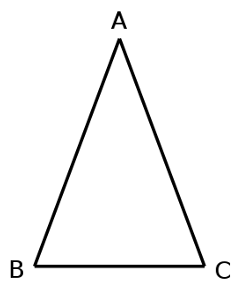# Self StudyHere’s an isosceles triangle. Sides AB and AC are equal, and this means that the angles opposite those sides are equal as well.

That’s intuitively reasonable, but proving it is tricky. Teachers of Euclid’s Elements came to call it the pons asinorum, or bridge of donkeys, because it was the first challenge that separated quick students from slow.

The simplest proof, attributed to Pappus of Alexandria, requires no additional construction at all. We know that two triangles are congruent if two sides and the included angle of one triangle are congruent to their corresponding parts in the other (the “side-angle-side” postulate). So Pappus suggested simply picking up the triangle above, flipping it over, and putting it down again, to produce a second triangle ACB. Now if we compare the respective parts of the two triangles, we find that angle A is equal to itself, AB = AC, and AC = AB. Thus the “left-hand” angles of the two triangles are congruent, and it follows that the base angles of the original triangle above are equal.

In his 1879 book Euclid and his Modern Rivals, Lewis Carroll accepts the proof but remarks that it “reminds one a little too vividly of the man who walked down his own throat.”